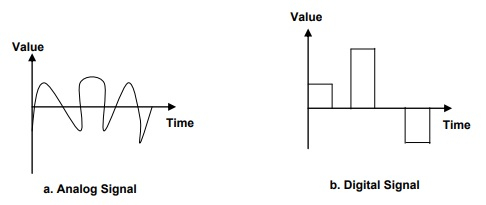# What are Analog and Digital signals?

Computer NetworkInternetMCA

#### Artificial Neural Network and Machine Learning using MATLAB

54 Lectures 4 hours

The physical layer’s major function is to move data in the form of electromagnetic signals over a transmission medium. The data which is used by a person is not in a form that can be transmitted over a network.

For example, a photograph has to be changed to a form that transmission media can accept. Transmission media works by conducting energy along a physical path.

Both data and the signals that represent them can be either Analog or digital in form.

## Analog Data

Analog data refers to information which is continuous.

For Example, an Analog clock which is having hour, minute, and second hands that gives information in a continuous form; the movement of the hands are continuous.

## Digital Data

Digital data refers to information which has discrete states.

For Example, a digital clock which reports the hours and minutes that will change suddenly from 10:05 to 10:06.

In this way the data can represent, signals can also represent either Analog or digital.

## Analog Signal

Analog signals have infinitely so many levels of intensity over a period of time. When the wave moves from value A to value B, it passes through and it includes an infinite number of values along its path.

## Digital Signal

Digital signals can have only a limited number of defined values. Although each value can be any number, it is often as simple as 0 or 1.

The easiest way to show signals is by plotting them on a pair of perpendicular axes.

• The vertical axis represents the value or strength of a signal.

• The horizontal axis represents time.The above figure shows the Analog signal and a digital signal. The curve represents the Analog signal through an infinite number of points. The vertical lines of the digital signal, however, demonstrate the sudden jump that the signal makes from value to value.